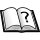Normal view

# Finite difference methods for ordinary and partial differential equations : steady-state and time-dependent problems / Randall J. LeVeque.

Material type:TextPublication details: Philadelphia, PA : Society for Industrial and Applied Mathematics, c2007.Description: xv, 341 p. : ill. ; 26 cmISBN:
• 9780898716290 (alk. paper)
Subject(s): DDC classification:
• 515.35 22 LEV
Contents:
Finite difference approximations -- Steady states and boundary value problems -- Elliptic equations -- Iterative methods for sparse linear systems -- The initial value problem for ordinary differential equations -- Zero-stability and convergence for initial value problems -- Absolute stability for ordinary differential equations -- Stiff ordinary differential equations -- Diffusion equations and parabolic problems -- Addiction equations and hyperbolic systems -- Mixed equations -- Appendixes: A. Measuring errors -- B. Polynomial interpolation and orthogonal polynomials -- C. Eigenvalues and inner-product norms -- D. Matrix powers and exponentials -- E. Partial differential equations.
Tags from this library: No tags from this library for this title.
Star ratingsAverage rating: 0.0 (0 votes)
Holdings
Item type Current library Collection Call number Copy number Status Notes Date due Barcode Item holdsReference IIT Goa Central Library Reference 515.35 / LEV (Browse shelf(Opens below)) 1 Reference TB1159||09-08-2023||25.00%||USD 79.50 4193
Total holds: 0

Includes bibliographical references (p. 329-335) and index.

Finite difference approximations -- Steady states and boundary value problems -- Elliptic equations -- Iterative methods for sparse linear systems -- The initial value problem for ordinary differential equations -- Zero-stability and convergence for initial value problems -- Absolute stability for ordinary differential equations -- Stiff ordinary differential equations -- Diffusion equations and parabolic problems -- Addiction equations and hyperbolic systems -- Mixed equations -- Appendixes: A. Measuring errors -- B. Polynomial interpolation and orthogonal polynomials -- C. Eigenvalues and inner-product norms -- D. Matrix powers and exponentials -- E. Partial differential equations.

Share